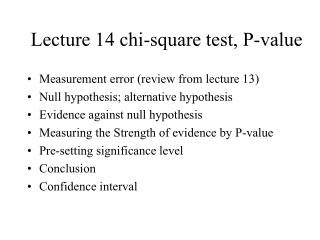DownloadDownload PresentationLecture 14 chi-square test, P-value

# Lecture 14 chi-square test, P-value

Télécharger la présentation## Lecture 14 chi-square test, P-value

- - - - - - - - - - - - - - - - - - - - - - - - - - - E N D - - - - - - - - - - - - - - - - - - - - - - - - - - -
##### Presentation Transcript

1. Lecture 14 chi-square test, P-value • Measurement error (review from lecture 13) • Null hypothesis; alternative hypothesis • Evidence against null hypothesis • Measuring the Strength of evidence by P-value • Pre-setting significance level • Conclusion • Confidence interval

2. Some general thoughts about hypothesis testing • A claim is any statement made about the truth; it could be a theory made by a scientist, or a statement from a prosecutor, a manufacture or a consumer • Data cannot prove a claim however, because there • May be other data that could contradict the theory • Data can be used to reject the claim if there is a contradiction to what may be expected • Put any claim in the null hypothesis H0 • Come up with an alternative hypothesis and put it as H1 • Study data and find a hypothesis testing statistics which is an informative summary of data that is most relevant in differentiating H1 from H0.

3. Testing statistics is obtained by experience or statistical training; it depends on the formulation of the problem and how the data are related to the hypothesis. • Find the strength of evidence by P-value : from a future set of data, compute the probability that the summary testing statistics will be as large as or even greater than the one obtained from the current data. If P-value is very small , then either the null hypothesis is false or you are extremely unlucky. So statistician will argue that this is a strong evidence against null hypothesis. If P-value is smaller than a pre-specified level (called significance level, 5% for example), then null hypothesis is rejected.

4. Back to the microarray example • Ho : true SD s=0.1 (denote 0.1 by s0) • H1 : true SD s > 0.1 (because this is the main concern; you don’t care if SD is small) • Summary : • Sample SD (s) = square root of ( sum of squares/ (n-1) ) = 0.18 • Where sum of squares = (1.1-1.3)2 + (1.2-1.3)2 + (1.4-1.3)2 + (1.5-1.3)2 = 0.1, n=4 • The ratio s/ s =1.8 , is it too big ? • The P-value consideration: • Suppose a future data set (n=4) will be collected. • Let s be the sample SD from this future dataset; it is random; so what is the probability that s/ will be • As big as or bigger than 1.8 ? P(s/ s0 >1.8)

5. P(s/ s0 >1.8) • But to find the probability we need to use chi-square distribution : • Recall that sum of squares/ true variance follow a chi-square distribution ; • Therefore, equivalently, we compute • P ( future sum of squares/ s02 > sum of squares from the currently available data/ s02), (recalls0 is • The value claimed under the null hypothesis) ;

6. Once again, if data were generated again, then Sum of squares/ true variance is random and follows a chi-squared distribution with n-1 degrees of freedom; where sum of squares= sum of squared distance between each data point and the sample mean Note : Sum of squares= (n-1) sample variance = (n-1)(sample SD)2 P-value = P(chi-square random variable> computed value from data)=P (chisquare random variable > 10.0) For our case, n=4; so look at the chi-square distribution with df=3; from table we see : P-value is between .025 and .01, reject null hypothesis at 5% significance level 9.348 11.34 The value computed from available data = .10/.01=10 (note sum of squares=.1, true variance =.12

7. Confidence interval • A 95% confidence interval for true variance s2 is • (Sum of squares/C2, sum of squares/C1) • Where C1 and C2 are the cutting points from chi-square table with d.f=n-1 so that • P(chisquare random variable > C1)= .975 • P(chisquare random variable>C2)=.025 • This interval is derived from • P( C1< sum of squares/ s2 <C2)=.95 For our data, sum of squares= .1 ; from d.f=3 of table, C1=.216, C2=9.348; so the confidence interval of s2 is 0.1017 to .4629; how about confidence interval of s ?